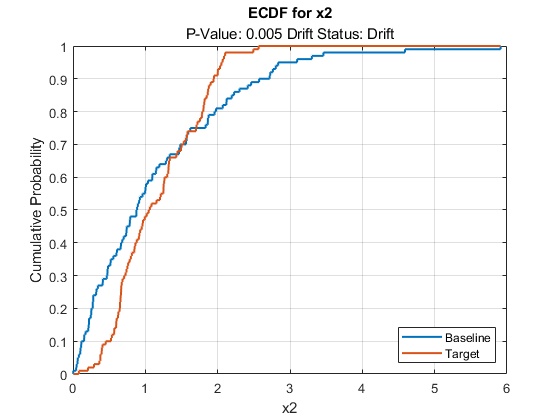# plotEmpiricalCDF

Plot empirical cumulative distribution function (ecdf) of a variable specified for data drift detection

## Syntax

``plotEmpiricalCDF(DDiagnostics)``
``plotEmpiricalCDF(DDiagnostics,Variable=variable)``
``plotEmpiricalCDF(ax,___)``
``St = plotEmpiricalCDF(___)``

## Description

example

````plotEmpiricalCDF(DDiagnostics)` plots the ecdf values of the baseline and target data for the continuous variable with the lowest p-value. If the data does not contain any continuous variables, then `plotEmpiricalCDF` does not generate a plot and, instead, returns a warning.If you set the value of `EstimatePValues` to `false` in the call to `detectdrift`, then `plotEmpiricalCDF` displays `NaN` for the p-value and the drift status.```

example

````plotEmpiricalCDF(DDiagnostics,Variable=variable)` plots the ecdf for the variable specified by `variable`.```

example

````plotEmpiricalCDF(ax,___)` plots on the axes `ax` instead of `gca`, using any of the input argument combinations in the previous syntaxes.```
````St = plotEmpiricalCDF(___)` plots the ecdf and returns an array of `Stair` objects `St`. Use this to inspect and modify the properties of the object. To learn more, see Stair Properties.```

## Examples

collapse all

Generate baseline and target data with three variables, where the distribution parameters of the second and third variables change for the target data.

```rng('default') % For reproducibility baseline = [normrnd(0,1,100,1),wblrnd(1.1,1,100,1),betarnd(1,2,100,1)]; target = [normrnd(0,1,100,1),wblrnd(1.2,2,100,1),betarnd(1.7,2.8,100,1)];```

Perform permutation testing for all variables to check for any drift between the baseline and target data.

`DDiagnostics = detectdrift(baseline,target)`
```DDiagnostics = DriftDiagnostics VariableNames: ["x1" "x2" "x3"] CategoricalVariables: [] DriftStatus: ["Stable" "Drift" "Warning"] PValues: [0.3850 0.0050 0.0910] ConfidenceIntervals: [2×3 double] MultipleTestDriftStatus: "Drift" DriftThreshold: 0.0500 WarningThreshold: 0.1000 Properties, Methods ```

Plot the ecdf for the variable with the lowest p-value.

`plotEmpiricalCDF(DDiagnostics)`By default, `plotEmpiricalCDF` plots the ecdf of the baseline and target data for the variable with the lowest p-value, which is `x2` in this case. You can see the difference between the two empirical cumulative distribution functions. The plot also displays the p-value and the drift status for variable `x2`.

Generate baseline and target data with three variables, where the distribution parameters of the second and third variables change for the target data.

```rng('default') % For reproducibility baseline = [normrnd(0,1,100,1),wblrnd(1.1,1,100,1),betarnd(1,2,100,1)]; target = [normrnd(0,1,100,1),wblrnd(1.2,2,100,1),betarnd(1.7,2.8,100,1)];```

Perform permutation testing for all variables to check for any drift between the baseline and target data.

`DDiagnostics = detectdrift(baseline,target)`
```DDiagnostics = DriftDiagnostics VariableNames: ["x1" "x2" "x3"] CategoricalVariables: [] DriftStatus: ["Stable" "Drift" "Warning"] PValues: [0.3850 0.0050 0.0910] ConfidenceIntervals: [2×3 double] MultipleTestDriftStatus: "Drift" DriftThreshold: 0.0500 WarningThreshold: 0.1000 Properties, Methods ```

Plot the ecdf for the third variable.

`plotEmpiricalCDF(DDiagnostics,Variable="x3")``plotEmpiricalCDF` plots the ecdf for the baseline and target data. The function also displays the estimated p-value and the drift status for the specified variable.

`load humanactivity`

For details on the data set, enter `Description` at the command line.

Assign the first 250 observations as baseline data and the next 250 as target data for columns 10 to 15.

```baseline = feat(1:250,10:15); target = feat(251:500,10:15);```

Test for drift on all variables.

`DDiagnostics = detectdrift(baseline,target)`
```DDiagnostics = DriftDiagnostics VariableNames: ["x1" "x2" "x3" "x4" "x5" "x6"] CategoricalVariables: [] DriftStatus: ["Drift" "Stable" "Stable" "Drift" "Stable" "Warning"] PValues: [1.0000e-03 0.5080 0.2370 1.0000e-03 0.5370 0.0820] ConfidenceIntervals: [2×6 double] MultipleTestDriftStatus: "Drift" DriftThreshold: 0.0500 WarningThreshold: 0.1000 Properties, Methods ```

The drift status for variables `x4` and `x6` is `Drift` and `Warning`, respectively. Plot the ecdf values for `x4` and `x6` in a tiled layout.

```tiledlayout(1,2); ax1 = nexttile; plotEmpiricalCDF(DDiagnostics,ax1,Variable="x4") ax2= nexttile; plotEmpiricalCDF(DDiagnostics,ax2,Variable="x6")```There is a greater difference between the ecdf of the baseline and target data for variable `x4`. The `detectdrift` function detects the shift for variable `x4`.

## Input Arguments

collapse all

Diagnostics of the permutation testing for drift detection, specified as a `DriftDiagnostics` object returned by `detectdrift`.

Variable for which to plot the ecdf, specified as a string, character vector, or integer index.

Example: `Variable="x3"`

Example: `Variable=3`

Data Types: `single` | `double` | `char` | `string`

Axes on which to plot, specified as an `Axes` or `UIAxes` object. If you do not specify `ax`, then `plotEmpiricalCDF` creates the plot using the current axes. For more information on creating an axes object, see `axes` and `uiaxes`.

## Version History

Introduced in R2022a